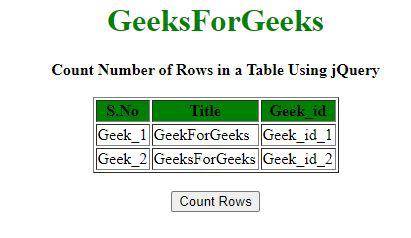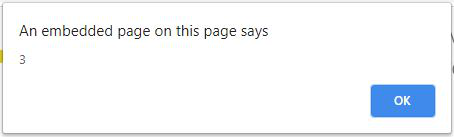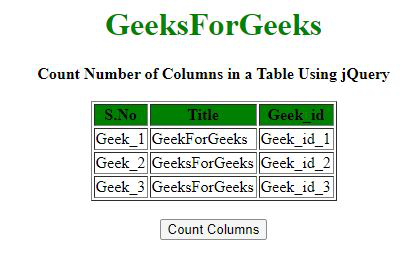Open in App
Not now

# How to Count Number of Rows and Columns in a Table Using jQuery?

• Difficulty Level : Basic
• Last Updated : 03 Aug, 2021

Given an HTML document containing a table, the task is to count the number of rows and columns in that table using JQuery.

Approach: The length property is used to count the number of rows and columns in an HTML table using jQuery. The selectors used for finding the number of rows and columns in an HTML table are:

• To count the number of rows, the “#Table_Id tr” selector is used. It selects all the <tr> elements in the table. This includes the row that contains the heading of the table. The length property is used on the selected elements to get the number of rows.
• To count the number of columns, the “#Table_Id tr th” selector is used. It selects all the number of <th> elements nested inside <tr> elements in the table. The length property is used on the selected elements to get the number of columns.

Example 1: In this example, the number of rows is counted.

## HTML

 ` ``<``html``> ``  ``<``head``> ``    ``<``title``> ``      ``Count Number of Rows and Columns in ``      ``a Table Using jQuery.``    `` `` ` `    ``<``script` `src` `= ``"https://ajax.googleapis.com/ajax/libs/jquery/3.4.0/jquery.min.js"``> ``    `` ``  `` `` ` `  ``<``body``> ``    ``<``center``>     ``      ``<``h1` `style` `= ``"color:green;"` `> ``        ``GeeksForGeeks ``      `` `` ` `      ``<``strong``> ``        ``Count Number of Rows in ``        ``a Table Using jQuery``      `` `` ` `      ``<``br``><``br``> `` ` `      ``<``table` `id``=``"Table_id"` `border``=``"1"` `width``=``"140"``>``        ``<``thead``>`` ` `          ``<``tr` `style` `= ``"background:green;"``> ``            ``<``th``>S.No ``            ``<``th``>Title ``            ``<``th``>Geek_id ``          `` ``        ````        ``<``tbody``>``          ``<``tr``> ``            ``<``td``>Geek_1 ``            ``<``td``>GeekForGeeks ``            ``<``td``>Geek_id_1 ``          `` ``          ``<``tr``> ``            ``<``td``>Geek_2 ``            ``<``td``>GeeksForGeeks ``            ``<``td``>Geek_id_2 ``          `` ``        ````      `` ``      ``<``br``> `` ` `      ``<``button` `type``=``"button"``> ``        ``Count Rows ``      `` `` ` `      ````      ``<``script``>``        ``\$(document).ready(function(){``          ``\$("button").click(function(){``             ` `            ``// Select all the rows in the table``            ``// and get the count of the selected elements``            ``var rowCount = \$("#Table_id tr").length;``            ``alert(rowCount); ``          ``});``        ``});``      `` ``    `` ``  `` ``                     `

Output:

• Before clicking on the button:• After clicking on the button:Example 2: In this example, the number of columns is counted.

## HTML

 ` ``<``html``> ``  ``<``head``> ``    ``<``title``> ``      ``Count Number of Rows and Columns in ``      ``a Table Using jQuery.``    `` `` ` `    ``<``script` `src` `= ``"https://ajax.googleapis.com/ajax/libs/jquery/3.4.0/jquery.min.js"``> ``    `` ``  `` `` ` `  ``<``body``> ``    ``<``center``>     ``      ``<``h1` `style` `= ``"color:green;"` `> ``        ``GeeksForGeeks ``      `` `` ` `      ``<``strong``> ``        ``Count Number of Columns in ``        ``a Table Using jQuery``      `` `` ` `      ``<``br``><``br``> `` ` `      ``<``table` `id``=``"Table_id"` `border``=``"1"` `width``=``"140"``>``        ``<``thead``>`` ` `          ``<``tr` `style` `= ``"background:green;"``> ``            ``<``th``>S.No ``            ``<``th``>Title ``            ``<``th``>Geek_id ``          `` ``        ````        ``<``tbody``>``          ``<``tr``> ``            ``<``td``>Geek_1 ``            ``<``td``>GeekForGeeks ``            ``<``td``>Geek_id_1 ``          `` ``          ``<``tr``> ``            ``<``td``>Geek_2 ``            ``<``td``>GeeksForGeeks ``            ``<``td``>Geek_id_2 ``          `` ``          ``<``tr``> ``            ``<``td``>Geek_3 ``            ``<``td``>GeeksForGeeks ``            ``<``td``>Geek_id_3 ``          `` ``        ````      `` ``      ``<``br``> `` ` `      ``<``button` `type``=``"button"``> ``        ``Count Columns ``      `` `` ` `      ````      ``<``script``>``        ``\$(document).ready(function(){``          ``\$("button").click(function(){``             ` `            ``// Select all the columns in the table``            ``// and get the count of the selected elements``            ``var colCount = \$("#Table_id tr th").length;``            ``alert(colCount); ``          ``});``        ``});``      `` ``    `` ``  `` ``                     `

Output:

• Before clicking on the button:• After clicking on the button:jQuery is an open source JavaScript library that simplifies the interactions between an HTML/CSS document, It is widely famous with it’s philosophy of “Write less, do more”.
You can learn jQuery from the ground up by following this jQuery Tutorial and jQuery Examples.

My Personal Notes arrow_drop_up